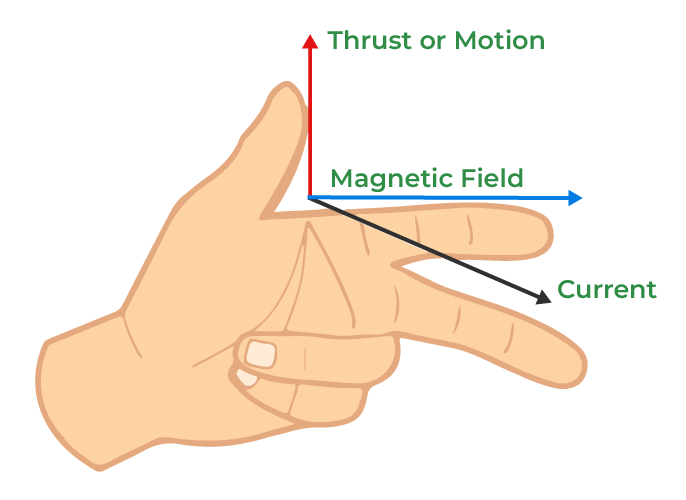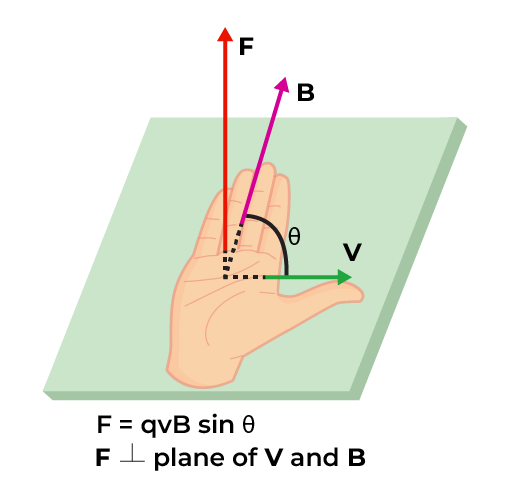GeeksforGeeks App
Open AppBrowser
Continue

# Force on a Current Carrying Conductor

Moving charges is an electric current that passes through a fixed point in a fixed period of time. Moving charges are responsible for establishing the magnetic field. The magnetic field is established due to the force exerted by the flow of moving charges.

When current is flowing through the conductor, a magnetic field is established around it. This phenomenon is called the magnetic effect of current.

## Lorentz Force

Force acting on a charge moving in the magnetic field is called Lorentz force. It is given by:

F = qvBsinθ

where
q is the charge,
v is the velocity of the charge moving in a magnetic field,
B is the magnetic field and
θ is the angle between the charge and magnetic field.

## Force on Current Carrying Conductor

When the current flows through the conductor kept in a magnetic field then the charges on the conductor are in motion so it experiences a force (Lorentz force). Let,

L is the length of the conductor,
I is current flowing through it,
q is the charges flowing through the conductor at the time ‘t’,
v is the velocity of the charge q,
B is a Uniform magnetic field in which current carrying conductor is placed

Then, according to Lorentz force

F = qvBsinθ                                   (Now, q = I × t, and v = L / t )

F = (I × t) × (L/t) × Bsinθ

F = ILBsinθ

where,
θ is the angle between L and B
L is the length of the conductor
I is current flowing through it
B is a Uniform magnetic field

### Vector Form

The vector form of the formula for finding force on the current-carrying conductor is given by,### Special Cases

A few special cases for finding force on the current carrying are,

Case I

When sin θ = 0 (minimum) i.e., θ = 0° or 180°, then force on the current element in a magnetic field is zero(minimum).

F = 0

A current element in a magnetic field does not experience any force if the current in it is collinear with the field.

Case II

When sin θ = 1 (maximum) i.e., θ = 90°, then force on the current element in a magnetic field is maximum (=ILB).

F = ILB

The direction of force is always perpendicular to the plane containingand## Fleming’s Left-Hand Rule

One of the important rules which are used in electrostatics is Fleming’s Left-hand Rule. It says if the forefinger, middle finger, and thumb of the left hand are stressed mutually perpendicular to each other such that the forefinger is along the direction of the magnetic field, the middle finger is along the direction of current then the thumb gives the direction of the force.## Right-Hand Palm Rule

Another important rule which is used in electrostatics is Right-Hand Palm Rule. It says if we, stretch the fingers and thumb of the right hand at right angles to each other. Then, if the fingers point in the direction of fieldand the thumb in the direction of the current I, the normal to palm will point in the direction of the force.## Solved Example of Force on Current Carrying Conductor

Example 1: A current of 1A flows in a wire of length 0.1cm in a magnetic field of 0.5T. Calculate the force acting on the wire when the wire makes an angle of (i) 90° (ii) 0° with respect to the magnetic field.

Solution:

(i) For an angle of 90°

F = ILBsinθ
= 1×0.1×0.5×sin90°
F = 0.05 N

(ii) For angle 0°

F = ILBsinθ
= 1×0.1×0.5×sin0°
F = 0N

Example 2: A current-carrying conductor of length 0.5cm with current 2A is placed at an angle of 30° in the magnetic field of 0.3T. Calculate the force acting on it.

Solution:

Given:

L = 0.5 cm, I = 2A, B = 0.3T and θ = 30°

F = ILBsinθ
F = 2×0.5×0.3×sin30°
= 0.3/2

F = 0.15N

Example 3: Find the length of the current-carrying conductor with 3A current which is placed at 90° in the magnetic field of 0.5T with 0.3 N force acting on it.

Solution:

Given:

I = 3A, B = 0.5T, F =0.3N and θ = 90°

F = ILBsinθ
0.3 = 3×L×0.5×sin90°
L = 0.3/1.5

L = 0.2cm

Example 4: At what angle the current-carrying conductor of length 0.6cm with a current 2A is placed in the magnetic field of 0.2T with 0.24N force acting on it?

Solution:

Given:

L = 0.6cm, I = 2A, B = 0.2T and F=0.24N

F = ILBsinθ
0.24 = 2×0.6×0.2×sinθ
1 = sinθ

θ = 90°

## FAQs on Force on Current Carrying Conductor

Question 1: State Fleming’s Left Hand Rule.

Fleming’s Left Hand Rule states that,

“Stretch the forefinger, central finger, and thumb of the left hand mutually perpendicular.Then if the forefinger points in the direction of the field (), the central finger in the direction of current I, the thumb will point in the direction of the force.”

Question 2: When the force acting on the current carrying conductor is maximum and state the equation of force.

The force acting on the current-carrying conductor is maximum when θ = 90° and the equation of force is

F = ILB.

Question 3: Write the vector form for the force acting on the current-carrying conductor.

Vector form for the force acting on the current-carrying conductor is given by:Question 4: When the force on the current-carrying conductor is zero.

When the angle between the length of the current-carrying conductor and the magnetic field is 0° i.e., both current-carrying conductors are collinear then force F =0.

Question 5: What is Lorentz force?